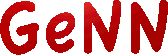GeNN  4.9.0 GPU enhanced Neuronal Networks (GeNN)
Brian interface (Brian2GeNN)

GeNN can simulate models written for the Brian simulator via the Brian2GeNN interface . In order for Brian2GeNN to find GeNN, GeNN must either be in your path with the location of the CUDA libraries configured via the `CUDA_PATH` environment variable (as described in Installation) or the `devices.genn.path` and `devices.genn.cuda_backend.cuda_path` Brian preferences should be set.

To use GeNN to simulate a Brian script, import the `brian2genn` package and switch Brian to the `genn` device. As an example, the following Python script will simulate Leaky-integrate-and-fire neurons with varying input currents to construct an f/I curve:

from brian2 import *
import brian2genn
set_device('genn')
n = 1000
duration = 1*second
tau = 10*ms
eqs = '''
dv/dt = (v0 - v) / tau : volt (unless refractory)
v0 : volt
'''
group = NeuronGroup(n, eqs, threshold='v > 10*mV', reset='v = 0*mV',
refractory=5*ms, method='exact')
group.v = 0*mV
group.v0 = '20*mV * i / (n-1)'
monitor = SpikeMonitor(group)
run(duration)

Of course, your simulation should be more complex than the example above to actually benefit from the performance gains of using a GPU via GeNN.